# Language Translation Using Deep Learning

This example shows how to train a German to English language translator using a recurrent sequence-to-sequence encoder-decoder model with attention.

Recurrent encoder-decoder models have proven successful at tasks such as abstractive text summarization and neural machine translation. These models consist of an encoder, which typically processes input data with a recurrent layer such as an LSTM layer, and a decoder which maps the encoded input into the desired output, typically also with a recurrent layer. Models that incorporate attention mechanisms into the models allow the decoder to focus on parts of the encoded input while generating the translation one time step at a time. This example implements Bahdanau attention  using the custom layer `attentionLayer`, attached to this example as a supporting file. To access this layer, open this example as a live script.

This diagram shows the structure of a language translation model. The input text, specified as a sequence of words, is passed through the encoder, which outputs an encoded version of the input sequence and a hidden state used to initialize the decoder state. The decoder makes predictions one word at a time using previous prediction as input and also outputs update state and context values.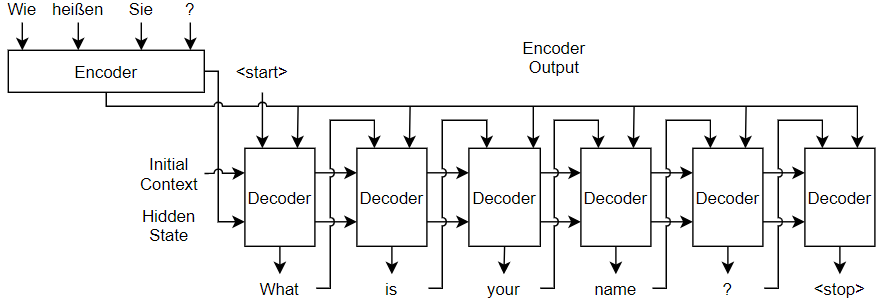For more information and the details about the encoder and decoder networks used in this example, see the Define Encoder and Decoder Networks section of the example.

Predicting the most likely word for each step in the sequence can lead to suboptimal results. Any incorrect predictions can cause even more incorrect predictions in later time steps. For example, for the target text `"An eagle flew by."`, if the decoder predicts the first word of a translation as `"A"`, then the probability of predicting "eagle" for the next word becomes much more unlikely because of the low probability of the phrase "a eagle" appearing in English text. The translation generation process differs for training and prediction. This example uses different approaches to stabilize training and the predictions:

• To stabilize training, you can randomly use the target values as inputs to the decoder. In particular, you can adjust the probability used to inject the target values as training progresses. For example, you can train using the target values at a much higher rate at the start of training, then decay the probability such that towards the end of training the model uses only the previous predictions. This technique is known as scheduled sampling . For more information, see the Decoder Predictions Function section of the example.

• To improve the predictions at translation time, for each time step, you can consider the top $K$ predictions for some positive integer $K$ and explore different sequences of predictions to identify the best combination. This technique is known as beam search. For more information, see the Beam Search Function section of the example.

This example shows how to load and preprocess text data to train a German to English language translator, define the encoder and decoder networks, train the model using a custom training loop, and generate translations using beam search.

Note: Language translation is a computationally intensive task. Training on the full data set used in this example can take many hours to run. To make the example run quicker, you can reduce training time at the cost of accuracy of predictions with previously unseen data by discarding a portion of the training data. Removing observations can speed up training because it reduces the amount of data to process in an epoch and reduces the vocabulary size of the training data.

To shorten the time it takes to run the example, discard 70% of the data. Note that discarding large amounts of data negatively affects the accuracy of the learned model. For more accurate results, reduce the amount of discarded data. To speed up the example, increase the amount of discarded data.

`discardProp = 0.70;`

```downloadFolder = tempdir; url = "http://www.manythings.org/anki/deu-eng.zip"; filename = fullfile(downloadFolder,"deu-eng.zip"); dataFolder = fullfile(downloadFolder,"deu-eng"); if ~exist(dataFolder,"dir") fprintf("Downloading English-German Tab-delimited Bilingual Sentence Pairs data set (7.6 MB)... ") websave(filename,url); unzip(filename,dataFolder); fprintf("Done.\n") end```

Create a table that contains the sentence pairs specified as strings. Read the tab-delimited sentences pairs using `readtable`. Specify the German text as the source and the English text as the target.

```filename = fullfile(dataFolder,"deu.txt"); opts = delimitedTextImportOptions(... Delimiter="\t", ... VariableNames=["Target" "Source" "License"], ... SelectedVariableNames=["Source" "Target"], ... VariableTypes=["string" "string" "string"], ... Encoding="UTF-8");```

View the first few sentence pairs in the data.

```data = readtable(filename, opts); head(data)```
```ans=8×2 table Source Target _______________ _______ "Geh." "Go." "Hallo!" "Hi." "Grüß Gott!" "Hi." "Lauf!" "Run!" "Lauf!" "Run." "Potzdonner!" "Wow!" "Donnerwetter!" "Wow!" "Feuer!" "Fire!" ```

Training on the full dataset can take a long time to run. To reduce training time at the cost of accuracy, you can discard a portion of the training data. Removing observations can speed up training because it reduces the amount of data to process in an epoch as well as reducing the vocabulary size of the training data.

Discard a portion of the data according to the `discardProp` variable defined at the start of the example. Note that discarding large amounts of data negatively affects the accuracy of the learned model. For more accurate results, reduce the amount of discarded data by setting `discardProp` to a lower value.

```idx = size(data,1) - floor(discardProp*size(data,1)) + 1; data(idx:end,:) = [];```

View the number of remaining observations.

`size(data,1)`
```ans = 68124 ```

Split the data into training and test partitions containing 90% and 10% of the data, respectively.

```trainingProp = 0.9; idx = randperm(size(data,1),floor(trainingProp*size(data,1))); dataTrain = data(idx,:); dataTest = data; dataTest(idx,:) = [];```

View the first few rows of the training data.

`head(dataTrain)`
```ans=8×2 table Source Target ___________________________________ _________________________ "Tom erschoss Mary." "Tom shot Mary." "Ruf mich bitte an." "Call me, please." "Kann das einer nachprüfen?" "Can someone check this?" "Das lasse ich mir nicht gefallen!" "I won't stand for it." "Ich mag Englisch nicht." "I don't like English." "Er ist auf dem Laufenden." "He is up to date." "Sie sieht glücklich aus." "She seems happy." "Wo wurden sie geboren?" "Where were they born?" ```

View the number of training observations.

`numObservationsTrain = size(dataTrain,1)`
```numObservationsTrain = 61311 ```

### Preprocess Data

Preprocess the text data using the `preprocessText` function, listed at the end of the example. The `preprocessText` function preprocesses and tokenizes the input text for translation by splitting the text into words and adding start and stop tokens.

`documentsGerman = preprocessText(dataTrain.Source);`

Create a `wordEncoding` object that maps tokens to a numeric index and vice versa using a vocabulary.

`encGerman = wordEncoding(documentsGerman);`

Convert the target data to sequences using the same steps.

```documentsEnglish = preprocessText(dataTrain.Target); encEnglish = wordEncoding(documentsEnglish);```

View the vocabulary sizes of the source and target encodings.

`numWordsGerman = encGerman.NumWords`
```numWordsGerman = 12117 ```
`numWordsEnglish = encEnglish.NumWords`
```numWordsEnglish = 7226 ```

### Define Encoder and Decoder Networks

This diagram shows the structure of a language translation model. The input text, specified as a sequence of words, is passed through the encoder, which outputs an encoded version of the input sequence and a hidden state used to initialize the decoder state. The decoder makes predictions one word at a time using previous the prediction as input and also outputs updated state and context values.Create the encoder and decoder networks using the `languageTranslationLayers` function, attached to this example as a supporting file. To access this function, open the example as a live script.

For the encoder network, the `languageTranslationLayers` function defines a simple network consisting of an embedding layer followed by an LSTM layer. An embedding operation converts categorical tokens into numeric vectors, where the numeric vectors are learned by the network.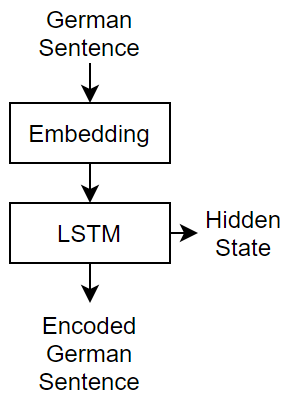For the decoder network, the `languageTranslationLayers` function defines a network that passes the input data concatenated with the input context through an LSTM layer, and takes the updated hidden state and the encoder output and passes it through an attention mechanism to determine the context vector. The LSTM output and the context vector are then concatenated and passed through a fully connected and a softmax layer for classification.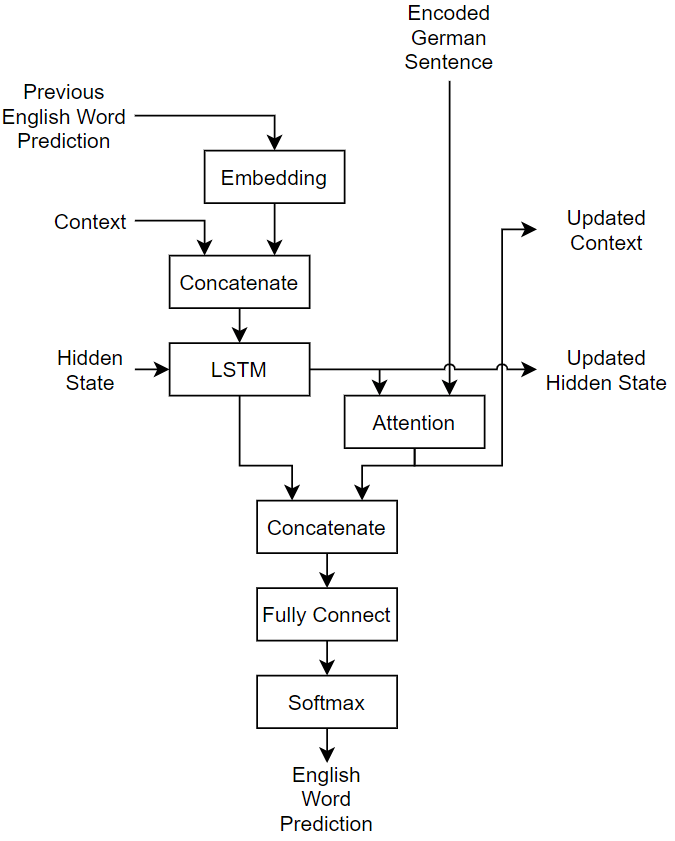Create the encoder and decoder networks using the `languageTranslationLayers` function, attached to this example as a supporting file. To access this function, open the example as a live script. Specify an embedding dimension of 128, and 128 hidden units in the LSTM layers.

```embeddingDimension = 128; numHiddenUnits = 128; [lgraphEncoder,lgraphDecoder] = languageTranslationLayers(embeddingDimension,numHiddenUnits,numWordsGerman,numWordsEnglish);```

To train the network in a custom training loop, convert the encoder and decoder networks to `dlnetwork` objects.

```dlnetEncoder = dlnetwork(lgraphEncoder); dlnetDecoder = dlnetwork(lgraphDecoder);```

The decoder has multiple outputs including the context output of the attention layer, which is also passed to another layer. Specify the network outputs using the `OutputNames` property of the decoder `dlnetwork` object.

`dlnetDecoder.OutputNames = ["softmax" "context" "lstm2/hidden" "lstm2/cell"];`

Create the function `modelGradients`, listed in the Model Gradients Function section of the example, which takes as input the encoder and decoder model parameters, a mini-batch of input data and the padding masks corresponding to the input data, and the dropout probability and returns the gradients of the loss with respect to the learnable parameters in the models and the corresponding loss.

### Specify Training Options

Train with a mini-batch size of 64 for 15 epochs and a learning rate of 0.005.

```miniBatchSize = 64; numEpochs = 15; learnRate = 0.005;```

Initialize the options for Adam optimization.

```gradientDecayFactor = 0.9; squaredGradientDecayFactor = 0.999;```

Train using gradually decaying values of $ϵ$ for scheduled sampling. Start with a value of $ϵ=0.5$ and linearly decay to end with a value of $ϵ=0$. For more information about scheduled sampling, see the Decoder Predictions Function section of the example.

```epsilonStart = 0.5; epsilonEnd = 0;```

Train using SortaGrad , which is a strategy to improve training of ragged sequences by training for one epoch with the sequences sorted by sequence then shuffling once per epoch thereafter.

Sort the training sequences by sequence length.

```sequenceLengths = doclength(documentsGerman); [~,idx] = sort(sequenceLengths); documentsGerman = documentsGerman(idx); documentsEnglish = documentsEnglish(idx);```

### Train Model

Train the model using a custom training loop.

Create array datastores for the source and target data using the `arrayDatastore` function. Combine the datastores using the `combine` function.

```adsSource = arrayDatastore(documentsGerman); adsTarget = arrayDatastore(documentsEnglish); cds = combine(adsSource,adsTarget);```

Create a mini-batch queue to automatically prepare mini-batches for training.

• Preprocess the training data using the `preprocessMiniBatch` function, which returns a mini-batch of source sequences, target sequences, the corresponding mask, and the initial start token.

• Output `dlarray` objects with the format `"CTB"` (channel, time, batch).

```mbq = minibatchqueue(cds,4, ... MiniBatchSize=miniBatchSize, ... MiniBatchFcn=@(X,Y) preprocessMiniBatch(X,Y,encGerman,encEnglish), ... MiniBatchFormat=["CTB" "CTB" "CTB" "CTB"], ... PartialMiniBatch="discard");```

Initialize the training progress plot.

```figure C = colororder; lineLossTrain = animatedline(Color=C(2,:)); xlabel("Iteration") ylabel("Loss") ylim([0 inf]) grid on```

For the encoder and decoder networks, initialize the values for Adam optimization.

```trailingAvgEncoder = []; trailingAvgSqEncoder = []; trailingAvgDecder = []; trailingAvgSqDecoder = [];```

Create an array of $ϵ$ values for scheduled sampling.

```numIterationsPerEpoch = floor(numObservationsTrain/miniBatchSize); numIterations = numIterationsPerEpoch * numEpochs; epsilon = linspace(epsilonStart,epsilonEnd,numIterations);```

Train the model. For each iteration:

• Read a mini-batch of data from the mini-batch queue.

• Compute the model gradients and loss.

• Update the encoder and decoder networks using the `adamupdate` function.

• Update the training progress plot and display an example translation using the `ind2str` function, attached to this example as a supporting file. To access this function, open this example as a live script.

• If the iteration yields the lowest training loss, then save the network.

At the end of each epoch, shuffle the mini-batch queue.

For large data sets, training can take many hours to run.

```iteration = 0; start = tic; lossMin = inf; reset(mbq) % Loop over epochs. for epoch = 1:numEpochs % Loop over mini-batches. while hasdata(mbq) iteration = iteration + 1; % Read mini-batch of data. [dlX,dlT,maskT,decoderInput] = next(mbq); % Compute loss and gradients. [gradientsEncoder,gradientsDecoder,loss,dlYPred] = dlfeval(@modelGradients,dlnetEncoder,dlnetDecoder,dlX,dlT,maskT,decoderInput,epsilon(iteration)); % Update network learnable parameters using adamupdate. [dlnetEncoder, trailingAvgEncoder, trailingAvgSqEncoder] = adamupdate(dlnetEncoder,gradientsEncoder,trailingAvgEncoder,trailingAvgSqEncoder, ... iteration,learnRate,gradientDecayFactor,squaredGradientDecayFactor); [dlnetDecoder, trailingAvgDecder, trailingAvgSqDecoder] = adamupdate(dlnetDecoder,gradientsDecoder,trailingAvgDecder,trailingAvgSqDecoder, ... iteration,learnRate,gradientDecayFactor,squaredGradientDecayFactor); % Generate translation for plot. if iteration == 1 || mod(iteration,10) == 0 strGerman = ind2str(dlX(:,1,:),encGerman); strEnglish = ind2str(dlT(:,1,:),encEnglish,Mask=maskT); strTranslated = ind2str(dlYPred(:,1,:),encEnglish); end % Display training progress. D = duration(0,0,toc(start),Format="hh:mm:ss"); loss = double(gather(extractdata(loss))); addpoints(lineLossTrain,iteration,loss) title( ... "Epoch: " + epoch + ", Elapsed: " + string(D) + newline + ... "Source: " + strGerman + newline + ... "Target: " + strEnglish + newline + ... "Training Translation: " + strTranslated) drawnow % Save best network. if loss < lossMin lossMin = loss; netBest.dlnetEncoder = dlnetEncoder; netBest.dlnetDecoder = dlnetDecoder; netBest.loss = loss; netBest.iteration = iteration; netBest.D = D; end end % Shuffle. shuffle(mbq); end```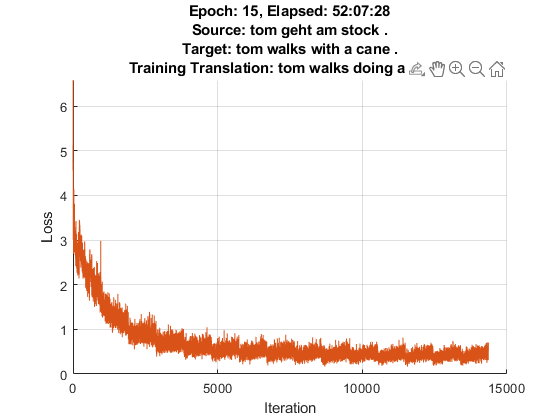The plot shows two translations of the source text. The target is the target translation provided by the training data that the network attempts to reproduce. The training translation is the predicted translation, which uses information from the target text via the scheduled sampling mechanism.

Add the word encodings to the `netBest` structure and save the structure in a MAT file.

```netBest.encGerman = encGerman; netBest.encEnglish = encEnglish; D = datestr(now,'yyyy_mm_dd__HH_MM_SS'); filename = "dlnet_best__" + D + ".mat"; save(filename,"netBest");```

Extract the best network from `netBest`.

```dlnetEncoder = netBest.dlnetEncoder; dlnetDecoder = netBest.dlnetDecoder;```

### Test Model

To evaluate the quality of the translations, use the BiLingual Evaluation Understudy (BLEU) scoring algorithm .

Translate the test data using the `translateText` function listed at the end of the example.

`strTranslatedTest = translateText(dlnetEncoder,dlnetDecoder,encGerman,encEnglish,dataTest.Source);`

View a random selection of the test source text, target text, and predicted translations in a table.

```numObservationsTest = size(dataTest,1); idx = randperm(numObservationsTest,8); tbl = table; tbl.Source = dataTest.Source(idx); tbl.Target = dataTest.Target(idx); tbl.Translated = strTranslatedTest(idx)```
```tbl=8×3 table Source Target Translated _____________________________________ __________________________ _________________________________ "Er sieht krank aus." "He seems ill." "he looks sick ." "Ich werde das Buch holen." "I'll get the book." "i'll get the book . . it . ." "Ruhst du dich jemals aus?" "Do you ever rest?" "do you look out of ? ? ? ?" "Was willst du?" "What are you after?" "what do you want want ? ? ? ?" "Du hast keinen Beweis." "You have no proof." "you have no proof . . . . ." "Macht es, wann immer ihr wollt." "Do it whenever you want." "do it you like it . . it ." "Tom ist gerade nach Hause gekommen." "Tom has just come home." "tom just came home home . . . ." "Er lügt nie." "He never tells a lie." "he never lie lies . . . . ." ```

To evaluate the quality of the translations using the BLEU similarity score, first preprocess the text data using the same steps as for training. Specify empty start and stop tokens, as these are not used in the translation.

```candidates = preprocessText(strTranslatedTest,StartToken="",StopToken=""); references = preprocessText(dataTest.Target,StartToken="",StopToken="");```

The `bleuEvaluationScore` function, by default, evaluates the similarity scores by comparing n-grams of length one through four (multiword phrases with four or fewer words or single words). If the candidate or reference documents have fewer than four tokens, then the resulting BLEU evaluation score is zero. To ensure that `bleuEvaluationScore` returns nonzero scores for these short candidate documents, set the n-gram weights to a vector with fewer elements than the number of words in `candidate`.

Determine the length of the shortest candidate document.

`minLength = min([doclength(candidates); doclength(references)])`
```minLength = 2 ```

If the shortest document has fewer than four tokens, then set the n-gram weights to a vector with a length matching the shortest document with equal weights that sum to one. Otherwise, specify n-gram weights of `[0.25 0.25 0.25 0.25]`. Note that if `minLength` is `1` (and consequently the n-gram weights is also `1`), then the `bleuEvaluationScore` function can return less meaningful results as it only compares individual words (unigrams) and does not compare any n-grams (multiword phrases).

```if minLength < 4 ngramWeights = ones(1,minLength) / minLength; else ngramWeights = [0.25 0.25 0.25 0.25]; end```

Calculate the BLEU evaluation scores by iterating over the translations and using the `bleuEvaluationScore` function.

```for i = 1:numObservationsTest score(i) = bleuEvaluationScore(candidates(i),references(i),NgramWeights=ngramWeights); end```

Visualize the BLEU evaluation scores in a histogram.

```figure histogram(score); title("BLEU Evaluation Scores") xlabel("Score") ylabel("Frequency")```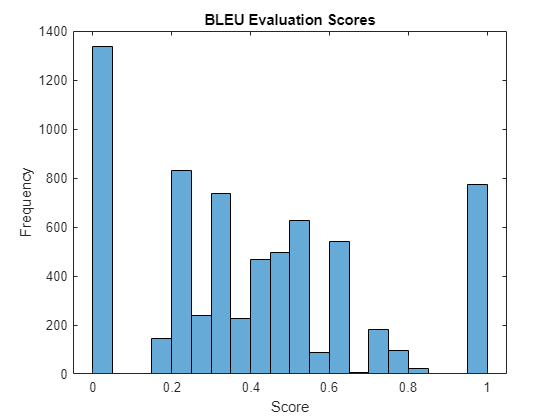View a table of some of the best translations.

```[~,idxSorted] = sort(score,"descend"); idx = idxSorted(1:8); tbl = table; tbl.Source = dataTest.Source(idx); tbl.Target = dataTest.Target(idx); tbl.Translated = strTranslatedTest(idx)```
```tbl=8×3 table Source Target Translated ________________________ ____________ _____________ "Legen Sie sich hin!" "Lie low." "lie low ." "Ich gähnte." "I yawned." "i yawned ." "Küsse Tom!" "Kiss Tom." "kiss tom ." "Küssen Sie Tom!" "Kiss Tom." "kiss tom ." "Nimm Tom." "Take Tom." "take tom ." "Komm bald." "Come soon." "come soon ." "Ich habe es geschafft." "I made it." "i made it ." "Ich sehe Tom." "I see Tom." "i see tom ." ```

View a table of some of the worst translations.

```idx = idxSorted(end-7:end); tbl = table; tbl.Source = dataTest.Source(idx); tbl.Target = dataTest.Target(idx); tbl.Translated = strTranslatedTest(idx)```
```tbl=8×3 table Source Target Translated _______________________________________________________________ __________________________ ____________________________ "Diese Schnecken kann man essen." "These snails are edible." "this can be eat ." "Sie stehen noch zu Verfügung." "They're still available." "it's still at . . . . . ." "Diese Schraube passt zu dieser Mutter." "This bolt fits this nut." "this life is too . . . . ." "Diese Puppe gehört mir." "This doll belongs to me." "this one is mine ." "Das ist eine japanische Puppe." "This is a Japanese doll." "that's a old trick ." "Das ist eine Kreuzung, an der alle Fahrzeuge anhalten müssen." "This is a four-way stop." "that's a to to to . . . ." "Diese Sendung ist eine Wiederholung." "This program is a rerun." "this is is quiet ." "Die heutige Folge ist eine Wiederholung." "Today's show is a rerun." "uranus is care ." ```

### Generate Translations

Generate translations for new data using the `translateText` function.

```strGermanNew = [ "Wie geht es Dir heute?" "Wie heißen Sie?" "Das Wetter ist heute gut."];```

Translate the text using the `translateText`, function listed at the end of the example.

`strTranslatedNew = translateText(dlnetEncoder,dlnetDecoder,encGerman,encEnglish,strGermanNew)`
```strTranslatedNew = 3×1 string "how do you feel today ?" "what's your your name ? ? ? ? ?" "the is is today . . today . ." ```

### Prediction Functions

#### Beam Search Function

Beam search is technique for exploring different combinations of time-step predictions to help find the best prediction sequence. The premise of beam search is for each time-step prediction, identify the top $K$ predictions for some positive integer $K$ (also known as the beam index or the beam width), and maintain the top $K$ predicted sequences so far at each time step.

This diagram shows the structure of an example beam search with beam index $K=3$. For each prediction, the top three sequences are maintained.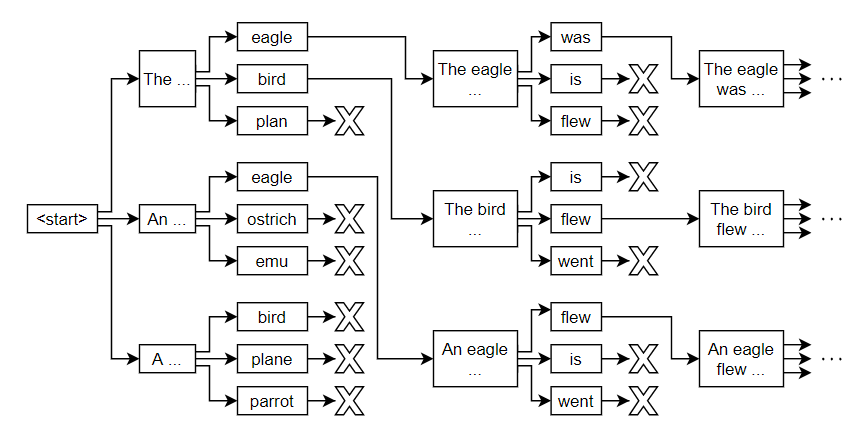The `beamSearch` function takes as input the input data `dlX`, the encoder and decoder networks, and the target word encoding, and returns the predicted translated words using the beam search algorithm with a beam index of 3 and a maximum sequence length of 10. You can also specify optional arguments using name-value arguments:

• `BeamIndex` — Beam index. The default is 3.

• `MaxNumWords` — Maximum sequence length. The default is 10.

```function str = beamSearch(dlX,dlnetEncoder,dlnetDecoder,encEnglish,args) % Parse input arguments. arguments dlX dlnetEncoder dlnetDecoder encEnglish args.BeamIndex = 3; args.MaxNumWords = 10; end beamIndex = args.BeamIndex; maxNumWords = args.MaxNumWords; startToken = "<start>"; stopToken = "<stop>"; % Encoder predictions. [dlZ, hiddenState, cellState] = predict(dlnetEncoder,dlX); % Initialize context. miniBatchSize = size(dlX,2); numHiddenUnits = size(dlZ,1); context = zeros([numHiddenUnits miniBatchSize],"like",dlZ); context = dlarray(context,"CB"); % Initialize candidates. candidates = struct; candidates.Words = startToken; candidates.Score = 0; candidates.StopFlag = false; candidates.HiddenState = hiddenState; candidates.CellState = cellState; % Loop over words. t = 0; while t < maxNumWords t = t + 1; candidatesNew = []; % Loop over candidates. for i = 1:numel(candidates) % Stop generating when stop token is predicted. if candidates(i).StopFlag continue end % Candidate details. words = candidates(i).Words; score = candidates(i).Score; hiddenState = candidates(i).HiddenState; cellState = candidates(i).CellState; % Predict next token. decoderInput = word2ind(encEnglish,words(end)); decoderInput = dlarray(decoderInput,"CBT"); [dlYPred,context,hiddenState,cellState] = predict(dlnetDecoder,decoderInput,hiddenState,cellState,context,dlZ, ... Outputs=["softmax" "context" "lstm2/hidden" "lstm2/cell"]); % Find top predictions. [scoresTop,idxTop] = maxk(extractdata(dlYPred),beamIndex); idxTop = gather(idxTop); % Loop over top predictions. for j = 1:beamIndex candidate = struct; % Determine candidate word and score. candidateWord = ind2word(encEnglish,idxTop(j)); candidateScore = scoresTop(j); % Set stop translating flag. if candidateWord == stopToken candidate.StopFlag = true; else candidate.StopFlag = false; end % Update candidate details. candidate.Words = [words candidateWord]; candidate.Score = score + log(candidateScore); candidate.HiddenState = hiddenState; candidate.CellState = cellState; % Add to new candidates. candidatesNew = [candidatesNew candidate]; end end % Get top candidates. [~,idx] = maxk([candidatesNew.Score],beamIndex); candidates = candidatesNew(idx); % Stop predicting when all candidates have stop token. if all([candidates.StopFlag]) break end end % Get top candidate. words = candidates(1).Words; % Convert to string scalar. words(ismember(words,[startToken stopToken])) = []; str = join(words); end```

#### Translate Text Function

The `translateText` function takes as input the encoder and decoder networks, an input string, and source and target word encodings and returns the translated text.

```function strTranslated = translateText(dlnetEncoder,dlnetDecoder,encGerman,encEnglish,strGerman,args) % Parse input arguments. arguments dlnetEncoder dlnetDecoder encGerman encEnglish strGerman args.BeamIndex = 3; end beamIndex = args.BeamIndex; % Preprocess text. documentsGerman = preprocessText(strGerman); dlX = preprocessPredictors(documentsGerman,encGerman); dlX = dlarray(dlX,"CTB"); % Loop over observations. numObservations = numel(strGerman); strTranslated = strings(numObservations,1); for n = 1:numObservations % Translate text. strTranslated(n) = beamSearch(dlX(:,n,:),dlnetEncoder,dlnetDecoder,encEnglish,BeamIndex=beamIndex); end end```

### Model Functions

The `modelGradients` function takes as input the encoder network, decoder network, mini-batches of predictors `dlX`, targets `dlT`, padding mask corresponding to the targets `maskT`, and $ϵ$ value for scheduled sampling. The function returns the gradients of the loss with respect to the learnable parameters in the networks `gradientsE` and `gradientsD`, the corresponding loss, and the decoder predictions `dlYPred` encoded as sequences of one-hot vectors.```function [gradientsE,gradientsD,loss,dlYPred] = modelGradients(dlnetEncoder,dlnetDecoder,dlX,dlT,maskT,decoderInput,epsilon) % Forward through encoder. [dlZ, hiddenState, cellState] = forward(dlnetEncoder,dlX); % Decoder output. dlY = decoderPredictions(dlnetDecoder,dlZ,dlT,hiddenState,cellState,decoderInput,epsilon); % Sparse cross-entropy loss. loss = sparseCrossEntropy(dlY,dlT,maskT); % Update gradients. [gradientsE,gradientsD] = dlgradient(loss,dlnetEncoder.Learnables,dlnetDecoder.Learnables); % For plotting, return loss normalized by sequence length. sequenceLength = size(dlT,3); loss = loss ./ sequenceLength; % For plotting example translations, return the decoder output. dlYPred = onehotdecode(dlY,1:size(dlY,1),1,"single"); end```

#### Decoder Predictions Function

The `decoderPredictions` function takes as input, the decoder network, the encoder output `dlZ`, the targets `dlT`, the decoder input hidden and cell state values, and the $ϵ$ value for scheduled sampling.

To stabilize training, you can randomly use the target values as inputs to the decoder. In particular, you can adjust the probability used to inject the target values as training progresses. For example, you can train using the target values at a much higher rate at the start of training, then decay the probability such that towards the end of training the model uses only the previous predictions. This technique is known as scheduled sampling . This diagram shows the sampling mechanism incorporated into one time step of a decoder prediction.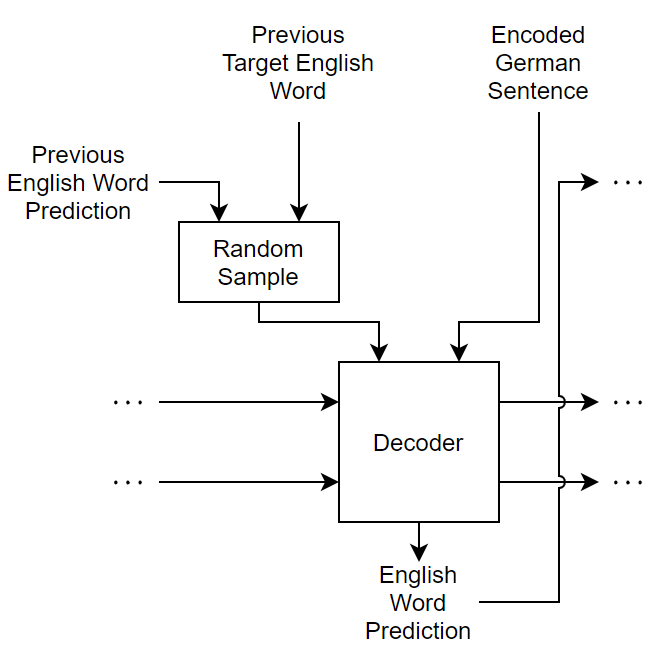The decoder makes predictions one time step at a time. At each time step, the input is randomly selected according to the $ϵ$ value for scheduled sampling. In particular, the function uses the target value as input with probability $ϵ$ and uses the previous prediction otherwise.

```function dlY = decoderPredictions(dlnetDecoder,dlZ,dlT,hiddenState,cellState,decoderInput,epsilon) % Initialize context. numHiddenUnits = size(dlZ,1); miniBatchSize = size(dlZ,2); context = zeros([numHiddenUnits miniBatchSize],"like",dlZ); context = dlarray(context,"CB"); % Initialize output. idx = (dlnetDecoder.Learnables.Layer == "fc" & dlnetDecoder.Learnables.Parameter=="Bias"); numClasses = numel(dlnetDecoder.Learnables.Value{idx}); sequenceLength = size(dlT,3); dlY = zeros([numClasses miniBatchSize sequenceLength],"like",dlZ); dlY = dlarray(dlY,"CBT"); % Forward start token through decoder. [dlY(:,:,1),context,hiddenState,cellState] = forward(dlnetDecoder,decoderInput,hiddenState,cellState,context,dlZ); % Loop over remaining time steps. for t = 2:sequenceLength % Scheduled sampling. Randomly select previous target or previous % prediction. if rand < epsilon % Use target value. decoderInput = dlT(:,:,t-1); else % Use previous prediction. [~,dlYhat] = max(dlY(:,:,t-1),[],1); decoderInput = dlYhat; end % Forward through decoder. [dlY(:,:,t),context,hiddenState,cellState] = forward(dlnetDecoder,decoderInput,hiddenState,cellState,context,dlZ); end end```

#### Sparse Cross-Entropy Loss

The `sparseCrossEntropy` function calculates the cross-entropy loss between the predictions `dlY` and targets `dlT` with the target mask `maskT`, where `dlY` is an array of probabilities and `dlT` is encoded as a sequence of integer values.

```function loss = sparseCrossEntropy(dlY,dlT,maskT) % Initialize loss. [~,miniBatchSize,sequenceLength] = size(dlY); loss = zeros([miniBatchSize sequenceLength],"like",dlY); % To prevent calculating log of 0, bound away from zero. precision = underlyingType(dlY); dlY(dlY < eps(precision)) = eps(precision); % Loop over time steps. for n = 1:miniBatchSize for t = 1:sequenceLength idx = dlT(1,n,t); loss(n,t) = -log(dlY(idx,n,t)); end end % Apply masking. maskT = squeeze(maskT); loss = loss .* maskT; % Calculate sum and normalize. loss = sum(loss,"all"); loss = loss / miniBatchSize; end```

### Preprocessing Functions

#### Text Preprocessing Function

The `preprocessText` function preprocesses the input text for translation by converting the text to lowercase, adding start and stop tokens, and tokenizing.

```function documents = preprocessText(str,args) arguments str args.StartToken = "<start>"; args.StopToken = "<stop>"; end startToken = args.StartToken; stopToken = args.StopToken; str = lower(str); str = startToken + str + stopToken; documents = tokenizedDocument(str,CustomTokens=[startToken stopToken]); end```

#### Mini-Batch Preprocessing Function

The `preprocessMiniBatch` function preprocesses tokenized documents for training. The function encodes mini-batches of documents as sequences of numeric indices and pads the sequences to have the same length.

```function [XSource,XTarget,mask,decoderInput] = preprocessMiniBatch(dataSource,dataTarget,encGerman,encEnglish) documentsGerman = cat(1,dataSource{:}); XSource = preprocessPredictors(documentsGerman,encGerman); documentsEngligh = cat(1,dataTarget{:}); sequencesTarget = doc2sequence(encEnglish,documentsEngligh,PaddingDirection="none"); [XTarget,mask] = padsequences(sequencesTarget,2,PaddingValue=1); decoderInput = XTarget(:,1,:); XTarget(:,1,:) = []; mask(:,1,:) = []; end```

#### Predictors Preprocessing Function

The `preprocessPredictors` function preprocesses source documents for training or prediction. The function encodes an array of tokenized documents as sequences of numeric indices.

```function XSource = preprocessPredictors(documentsGerman,encGerman) sequencesSource = doc2sequence(encGerman,documentsGerman,PaddingDirection="none"); XSource = padsequences(sequencesSource,2); end```

### Bibliography

1. Chorowski, Jan, Dzmitry Bahdanau, Dmitriy Serdyuk, Kyunghyun Cho, and Yoshua Bengio. “Attention-Based Models for Speech Recognition.” Preprint, siubmitted June 24, 2015. https://arxiv.org/abs/1506.07503.

2. Bengio, Samy, Oriol Vinyals, Navdeep Jaitly, and Noam Shazeer. “Scheduled Sampling for Sequence Prediction with Recurrent Neural Networks.” Preprint, submitted September 23, 2015. https://arxiv.org/abs/1506.03099.

3. Amodei, Dario, Sundaram Ananthanarayanan, Rishita Anubhai, Jingliang Bai, Eric Battenberg, Carl Case, Jared Casper et al. "Deep Speech 2: End-to-End Speech Recognition in English and Mandarin." In <cite>Proceedings of Machine Learning Research</cite> 48 (2016): 173–182.

4. Papineni, Kishore, Salim Roukos, Todd Ward, and Wei-Jing Zhu. "BLEU: A Method for Automatic Evaluation of Machine Translation." In Proceedings of the 40th Annual Meeting on Association for Computational Linguistics (2002): 311–318.